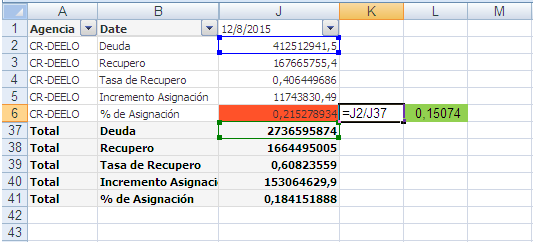# QlikView App Development

Discussion Board for collaboration related to QlikView App Development.

HighlightedNot applicable

## How to calculate % divided by total in pivot and set analysis

Hi!I try funtion in "% de Asignación"

=sum( {\$<recd_tramo ={'1','2'}  >}  recd_deuda_total)

/

sum(TOTAL  < recd_ccc_ajustado >{\$<recd_tramo ={'1','2'}  >}  recd_deuda_total  )

Thanks!!

Tags (2)
1 Solution

Accepted SolutionsMVP

## Re: How to calculate % divided by total in pivot and set analysis

I think you need Date in your TOTAL qualifier field list:

=sum( {\$<recd_tramo ={'1','2'}  >}  recd_deuda_total)

/

sum(TOTAL  < Date >{\$<recd_tramo ={'1','2'}  >}  recd_deuda_total  )